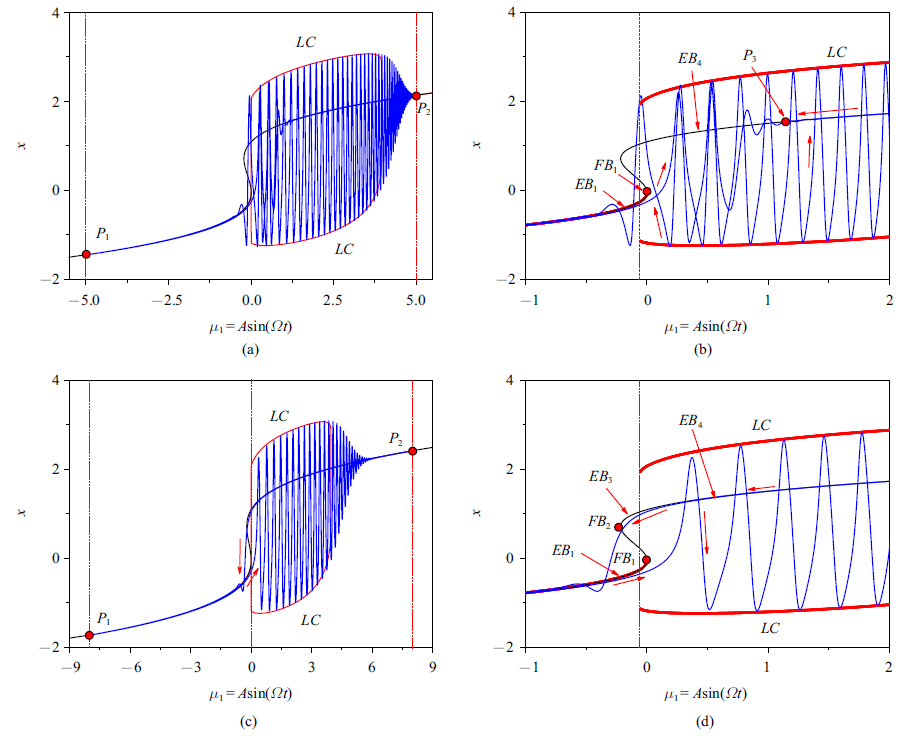余维三fold-fold-Hopf分岔下簇发振荡及其分类1) 薛淼, 葛亚威, 张正娣, 毕勤胜 BURSTING OSCILLATIONS AS WELL AS THE CLASSIFICATION IN THE FIELD WITH CODIMENSION-3 FOLD-FOLD-HOPF BIFURCATION1) Xue Miao, Ge Yawei, Zhang Zhengdi, Bi Qinsheng 图16 (a) $A=5.0,\mu_{2} =0.1$时转换相图和平衡曲线$(\mu_{1},x)$平面上的叠加图; (b) (a)图的局部放大图; (c) $A=8.0$, $\mu_{2}=0.1$时转换相图和平衡曲线$(\mu_{1} ,x)$平面上的叠加图; (d) (c)图的局部放大图 Fig.16 (a) Overlap of the transformed phase portrait and equilibrium branches on the $(\mu_{1} ,x)$ plane for $A=5.0,\mu_{2} =0.1$; (b) locally enlarged parts in (a); (c) overlap of the transformed phase portrait and equilibrium branches on the $(\mu_{1} ,x)$ plane for $A=8.0,\mu_{2} =0.1$; (d) locally enlarged parts in (c)